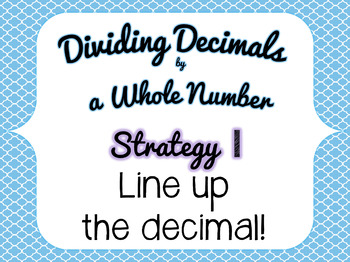# Dividing Decimals Powerpoint

Rated 4.85 out of 5, based on 382 reviews
382 RatingsSubjects
Resource Type
Formats Included
• PPTX
Pages
88 pages
Report this resource to TPT

### Description

Vocabulary, Foldables, and Instructions for Dividing a Decimal by a Whole Number and Dividing a Whole Number by a Decimal. Updated to include a Beat the Clock game for each skill. Students answer quickly to trying to answer before the computer does.

**Updates From 53 slides to 88 slides! More practice slides added. Now featuring Beat the Clock: the students try to answer the problem before the computer does. I wanted to update the look. I changed the background and updated the fonts on some key slides. When I did this I included some slides that can now be printed as posters. I also updated the foldable.**

I used this Powerpoint in my classroom over two days.

On the first day I focused on Dividing a Decimal by a Whole Number using decimal alignment as a strategy. I also reviewed basic vocabulary needed for division including divisor, dividend, and quotient.

On the second day we dealt with the challenging concept of Dividing a Whole Number by a Decimal.

The Powerpoint goes breaks each topic into step by step instructions and allows the students to attempt the skill on their paper, white board, or on the provided foldables. Two Foldables can be printed from the Powerpoint so students can keep track of the steps, attempt and example, and write down the strategy.

I wrote tips about using the foldable in your Interactive Math Notebooks, aka Math Journal, in the notes of the foldable slides.

The last few slides have a chant that can help students remember when to use the two strategies. I printed the last slide to put up on my math wall.

I showed this Powerpoint on my Smart Board.

Do you have Google Accounts for your students? Consider the digital interactive version: Dividing Decimals: Interactive Math for the Google Classroom

Fifth Grade Common Core Standard Mathematics Number & Operations in Base Ten 5.NBT.2 Explain patterns in the number of zeros of the product when multiplying a number by powers of 10, and explain patterns in the placement of the decimal point when a decimal is multiplied or divided by a power of 10. Use whole number
exponents to denote powers of 10.

Fifth Grade Common Core Standard Mathematics Number & Operations in Base Ten 5.NBT.7
Add, subtract, multiply, and divide decimals to hundredths, using concrete models or drawings and strategies based on place value, properties of operations, and/or the relationship between addition and subtraction; relate the strategy to a written method and explain the reasoning used.

Sixth Grade Common Core StandardMathematicsThe Number System6.NS.3Fluently add, subtract, multiply, and divide multi-digit decimals using
the standard algorithm for each operation.

Check Out My Other Math Products:

Find all my Division Products.

Order of Operations PEMDAS Packet

Equivalent Fractions: Game, Vocabulary, and More (Common Core Resource)

Factoring: Factor Trees, Prime Factorization Games, Foldable, and More

Prime, Composite, or Neither? Game, Foldable, and More

Plot a Point in Quadrant 1 (Common Core Resource)
Total Pages
88 pages